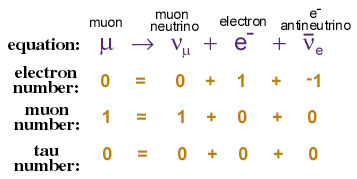Lepton type conservation

Leptons are divided into three lepton families: the electron and its neutrino, the muon and its neutrino, and the tau and its neutrino.

We use the terms "electron number," "muon number," and "tau number" to refer to the lepton family of a particle. Electrons and their neutrinos have electron number +1, positrons and their antineutrinos have electron number -1, and all other particles have electron number 0. Muon number and tau number operate analogously with the other two lepton families.

One important thing about leptons, then, is that electron number, muon number, and tau number are always conserved when a massive lepton decays into smaller ones.

### Let's take an example decay.

A muon decays into a muon neutrino, an electron, and an electron antineutrino:As you can see, electron, muon, and tau numbers are conserved. These and other conservation laws are what we believe define whether or not a given hypothetical lepton decay is possible.